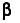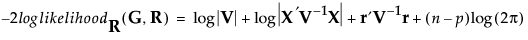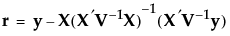Option Description Reference Fit Statistics Shows report for model fit statistics. Fit Statistics Random Effects Covariance Parameter Estimates Shows report of random effects covariance parameter estimates. This report appears when you specify random effects in the launch window. Random Effects Covariance Parameter Estimates Fixed Effects Parameter Estimates Shows report of fixed effects parameter estimates. Fixed Effects Parameter Estimates Repeated Effects Covariance Parameter Estimates Shows report of repeated effects covariance parameter estimates. This report appears when you specify repeated effects in the launch window. Repeated Effects Covariance Parameter Estimates Random Coefficients Shows report of random coefficients. This report appears when you specify random effects in the launch window. Random Coefficients Random Effects Predictions Shows report of random effect predictions. This report appears when you specify random effects in the launch window. Random Effects Predictions Fixed Effects Test Shows tests of fixed effects. This report appears when you specify fixed effects in the launch window. Fixed Effects Tests
Description of Fit Statistics Report uses the following notation:
 • Write the mixed model as follows:
Here y is the nx1 vector of observations,is a vector of fixed-effect parameters, γ is a vector of random-effect parameters, and ε is a vector of errors.
 • The vectors γ and ε are assumed to have a multivariate normal distribution where
 • With these assumptions, the variance of y is given as follows:
 -2 Residual Log Likelihood The final evaluation of twice the negative residual log likelihood, the objective function.whereand p is the rank of X. Use the residual likelihood only for model comparisons where the fixed effects portion of the model is identical. For more details, see Likelihood, AICc, and BIC in Statistical Details. -2 Log Likelihood Use the log likelihood for model comparisons in which the fixed, random, and repeated effects differ in any of the models. AICc BIC
If there are problems with model convergence, a warning message is displayed below the fit statistics. Convergence Score Test shows the warning that suggests the cause and possible solutions to the convergence issue. It also includes a test of the relative gradient at the final iteration. If this test is non-significant, the model might be correct but not fully reaching the convergence criteria. In this case, consider using the model and results with caution. For details, see Convergence Score Test.
Convergence Score Test
 Covariance Parameter Lists all the covariance parameters of the random effects that you specified in the model. Subject Lists the subject from which the block diagonal covariance matrix was formed. Estimate Gives the estimated variance or covariance component for the effect. Std Error Gives the standard error for the covariance component estimate. 95% Lower 95% Upper
 • the estimate
 • the standard error (Std Error)
 • a t test for the hypothesis that the estimate equals zero
 • a 95% confidence interval on the estimate
 Term Estimate Gives the parameter estimate for each term. This is the estimate of the term’s coefficient in the model. Std Error Gives an estimate of the standard error for the parameter estimate. DFDen t Ratio Tests whether the true value of the parameter is zero. The t Ratio is the ratio of the estimate to its standard error. Given the usual assumptions about the model, the t Ratio has a Student’s t distribution under the null hypothesis. Prob>|t| Lists the p-value for a two-sided test of the t Ratio. 95% Lower Shows the lower 95% confidence limit for the parameter. 95% Upper Shows the upper 95% confidence limit for the parameter.
 Covariance Parameter Lists all the covariance parameters for the repeated effects in the model. Estimate Gives the estimated variance or covariance component for the effect. Std Error Gives the standard error for the variance or covariance component estimate. 95% Lower 95% Upper
For each random effect in the model, this report gives an estimate known as the best linear unbiased predictor (BLUP), its standard error, and a Wald-based confidence interval.
This report shows a significance test for each fixed effect in the model. The test for a given effect tests the null hypothesis that all parameters associated with that effect are zero. An effect might have only one parameter as for a single continuous explanatory variable. In this case, the test is equivalent to the t test for that term in the Fixed Effects Parameter Estimates report. A nominal or ordinal effect can have several associated parameters, based on its number of levels. The effect test for such an effect tests whether all of the associated parameters are zero.
 Source Lists the fixed effects in the model. Nparm Shows the number of parameters associated with the effect. A continuous effect has one parameter. The number of parameters for a nominal or ordinal effect is one less than its number of levels. The number of parameters for a crossed effect is the product of the number of parameters for each individual effect. DFNum Shows the numerator degrees of freedom for the effect test. DFDen F Ratio Gives the computed F ratio for testing that the effect is zero. Prob > F Gives the p-value for the effect test.